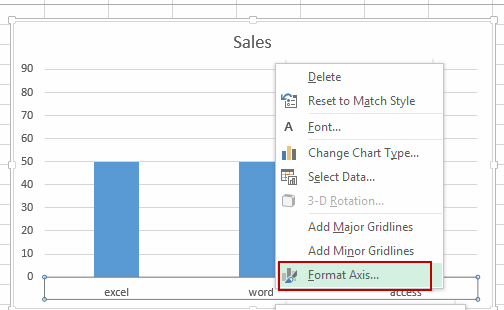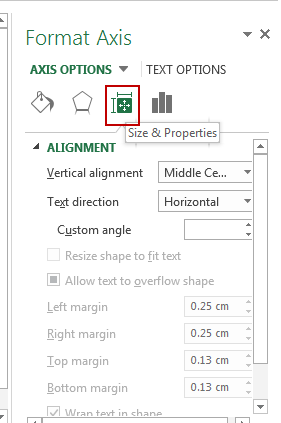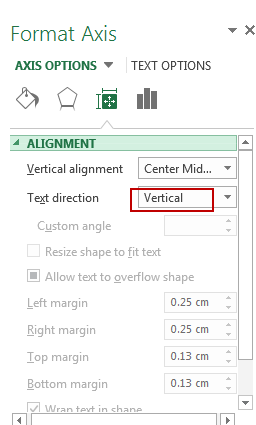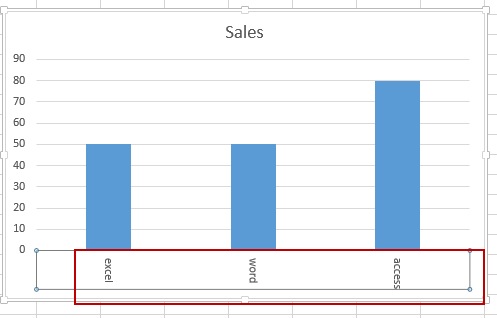# Rotate Axis labels

This post will guide you how to rotate axis labels in Excel 2007/2003/2016. How do I change the text direction of the vertical axis label to rotate all text 270 in Chart in Excel. How to rotate X Axis labels in Chart in Microsoft Excel 2013.

## Rotate Axis labels

In Excel chart, Axis labels are displayed horizontally, if you want to change X Axis Labels direction from horizontal to vertical. How to achieve it. Just do the following steps:

#1 right click on the X Axis label, and select Format Axis from the popup menu list. And the Format Axis pane will open.# 2 click the Size & Properties button in the Format Axis pane.#3 click Text direction list box, and choose Vertical from the drop down list box. Then close the Format Axis pane.#4 the X Axis text has been rotated from horizontal to vertical.For Excel 2007/2010:

You need to do the below steps to rotate Axis labels:

#1 right click on the X Axis label, and select Format Axis from the popup menu list. And the Format Axis dialog will open.

x
How to Create Dynamic Interactive Charts in Excel

#2 click Alignment menu in the left of Format Axis dialog. And select Rotate all text 90 from the Text direction drop down list box. And click Close button to close the Format Axis dialog.

Related Posts

If Cell is This Value or That Value

IF function is frequently used in Excel worksheet to return you expect “true value” or “false value” based on the result of logical test. If you want to see if a cell is A or B, and if one of ...

If Value is Greater Than A Certain Value

IF function is frequently used in Excel worksheet to return you expect “true value” or “false value” based on the logical test result. If you want to see if a value in one cell is greater than a specific value, ...

If Cell is Not Blank

IF function is frequently used in Excel worksheet to return you expect “true value” or “false value” based on the result of created logical test. If you want to see if a cell is blank or not, and leave some ...

VBA Macro For VLOOKUP From Another Sheet

In the previous post, you should know that how to fix or remove the #N/A error when using VLOOKUP formula to lookup value from another sheet. And this post will show you how to use VBA code to vlookup data ...

If Cell is Blank

IF function is frequently used in Excel worksheet to return you expect “true value” or “false value” based on the result of created logical test. If you want to see if a cell is blank or not, and leave some ...

If Cell Equals Certain Text String

IF function is frequently used in Excel worksheet to return you expect “true value” or “false value” based on the result of created logical test. If you want to see if cell equals a certain text string like “Win”, you ...

If Cell Contains Either Text1 or Text2

IF function is frequently used in Excel worksheet to return “true value” or “false value” based on the logical test result. If you want to see if cell contains certain substring1 like “abc” or substring2 like “def”, and returns true ...

If Cell Contains Certain Text OR Equals Certain Text

IF cell equals certain text IF function is frequently used in Excel worksheet to return “true value” or “false value” based on the logical test result. If you want to test values to see if they equal certain text like ...

VLOOKUP From Another Sheet Not Working

In the previous post, you should know that how to fix or remove the #N/A error when using VLOOKUP formula to lookup value from another sheet. And this post will show you reasons why your VLOOKUP formula is not working ...

If Cell Begins with One of Three Supplied Characters

If you want to test values to see if they begin with some given specific characters like “x”, ”y”, or “z”, you can create a formula with COUNTIF and SUM functions to return results. EXAMPLE You can see “TRUE” or ...

Sidebar